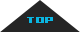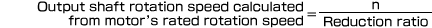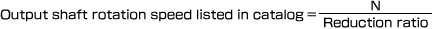FAQ

Q
Why does the output shaft rotation speed listed in the catalog differ from the output shaf

Q: Why does the output shaft rotation speed listed in the catalog differ from the output shaft rotation speed obtained by dividing the motor's rated rotation speed by the reduction ratio?

A: The output shaft rotation speed listed in the catalog is obtained by dividing the rotation speed N of the rotating magnetic field by the reduction ratio. For this reason, these numbers serve as the designated rotation speed and differ from the actual observed speed. Rotation speed data in the catalog is intended to serve as an aid in the selection of a reduction ratio.

Motor (induction motor) rotation speed:n

The rotation speed of an induction motor must be delayed somewhat relative to the rotating magnetic field created by the stator. This characteristic can be expressed as the following formula:

n =(1-S)×N

N : Rotation speed of rotating magnetic field
(Reference) For a 4-pole motor, 50Hz: N = 1,500 rpm; 60Hz: N = 1,800 rpm
S : Slip ratio (0<S<1)

The relationship of n and N in the above formula provides the reason for the difference in the output shaft rotation speeds.*Motor rated rotation speed
The motor rated rotation speed indicates the rotation speed when the motor is operated at its rated output and voltage.

Design by e-work